Question

# For an unknown block submerged in water, you determine the buoyance force on the block is...

For an unknown block submerged in water, you determine the buoyance force on the block is 5.4N. What is the volume (in unit of cm3) of the object?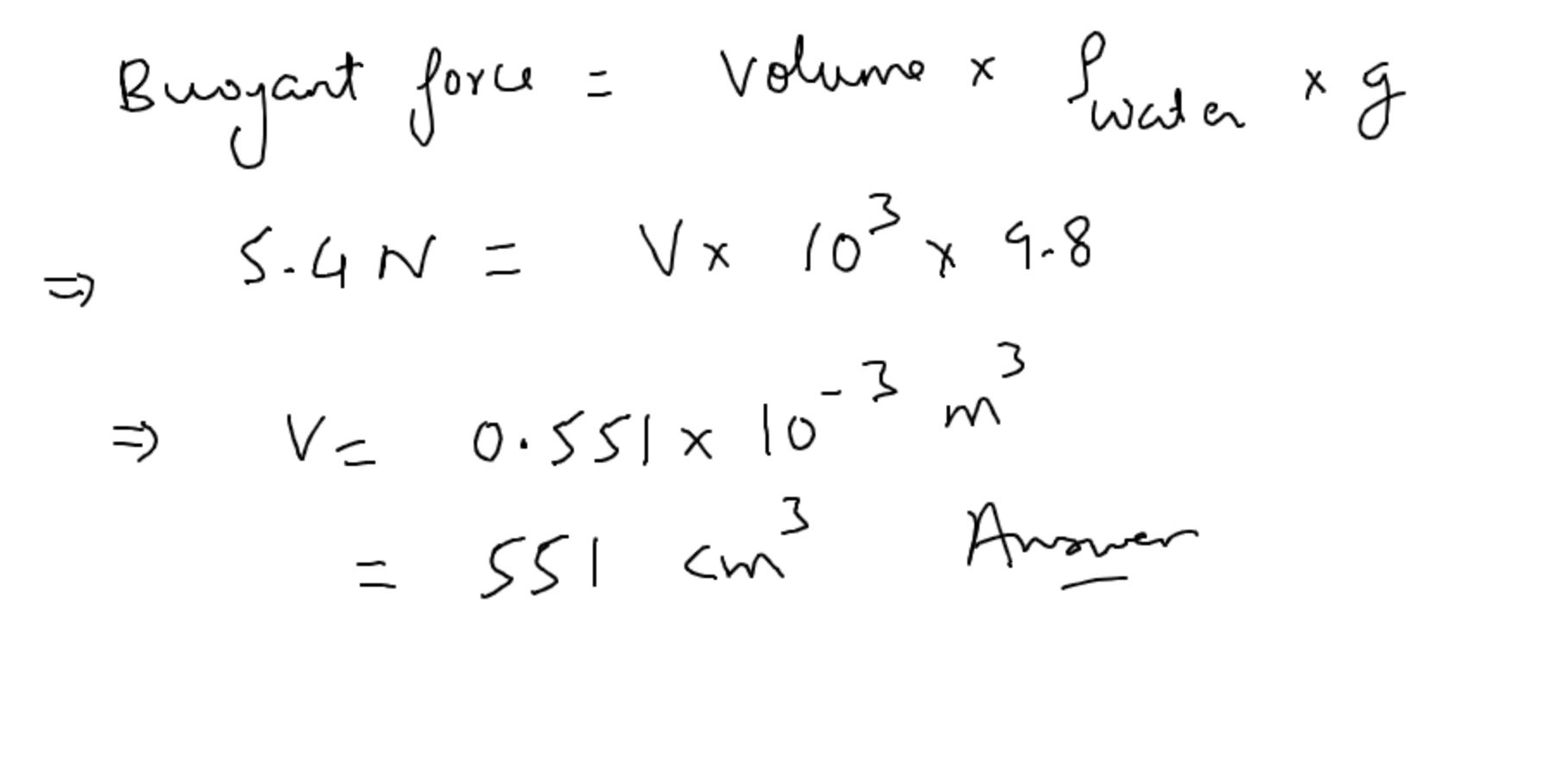#### Earn Coins

Coins can be redeemed for fabulous gifts.

Similar Homework Help Questions
• ### 36. A 100-cm' block is submerged in water. What is the buoyant force on the block...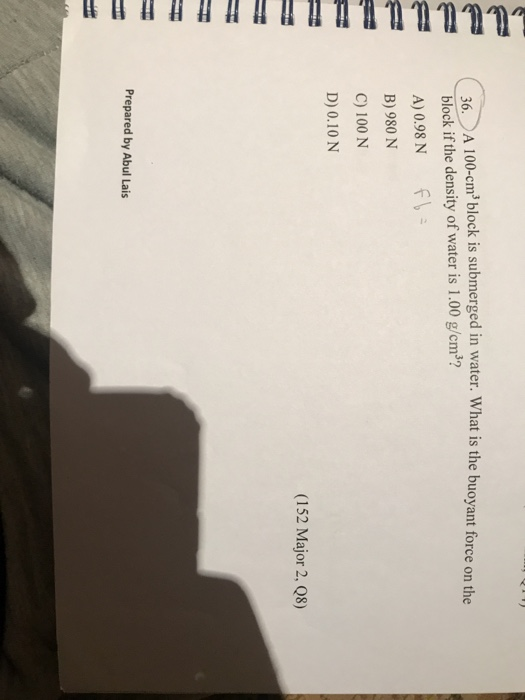36. A 100-cm' block is submerged in water. What is the buoyant force on the block if the density of water is 1.00 g/cm"? A) 0.98 N F B) 980 N C) 100 N D) 0.10 N (152 Major 2, 08) Prepared by Abul Lais

• ### 2. 95% of a wood block is submerged in water (density= 1000 kg/m). A layer of...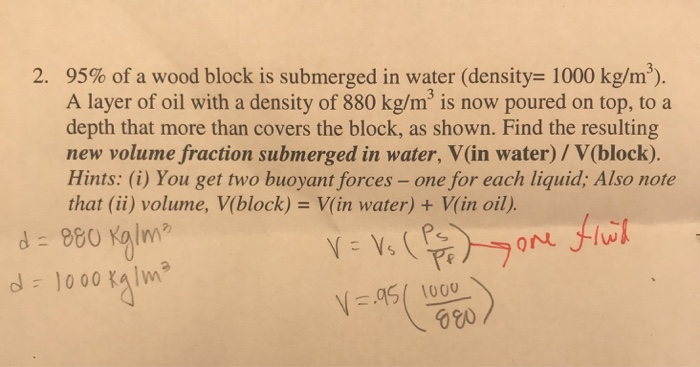2. 95% of a wood block is submerged in water (density= 1000 kg/m). A layer of oil with a density of 880 kg/m' is now poured on top, to a depth that more than covers the block, as shown. Find the resulting new volume fraction submerged in water, V(in water) / V(block). Hints: (i) You get two buoyant forces - one for each liquid; Also note that (ii) volume, V(block) = V(in water) + V(in oil). d=880 kg/m² V =...

• ### An object that weighs 1000 N floats with 80% of its volume submerged below water. What...

An object that weighs 1000 N floats with 80% of its volume submerged below water. What is the boyance force? What is the volume of the object?

• ### QUESTION 10 A block is submerged in water as shown the figure. Calculate the the volume...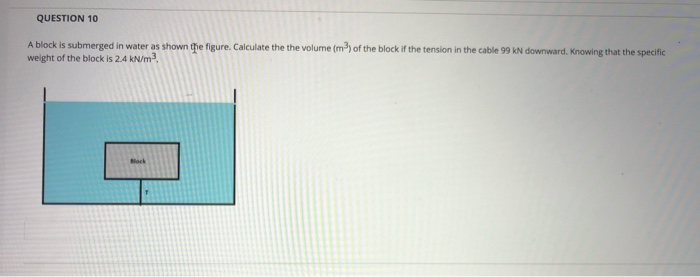QUESTION 10 A block is submerged in water as shown the figure. Calculate the the volume (m) of the block if the tension in the cable 99 kN downward. Knowing that the specific weight of the block is 2.4 kN/m2 Block

• ### QUESTION 9 5p A block is submerged in water as shown the figure. Calculate the the...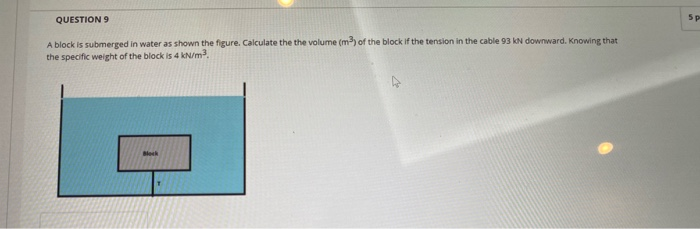QUESTION 9 5p A block is submerged in water as shown the figure. Calculate the the volume (m2) of the block if the tension in the cable 93 kN downward. Knowing that the specific weight of the block is 4 kN/m? Block

• ### Using Figures A and B, find the volume of the object submerged in the water and...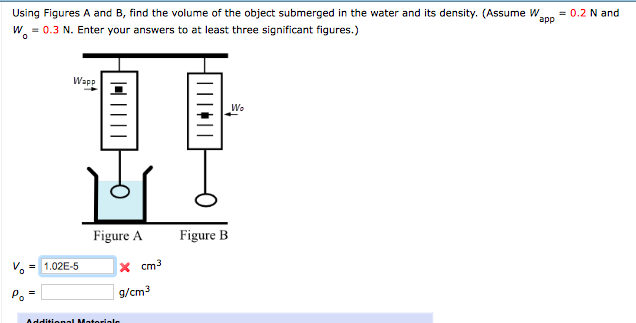Using Figures A and B, find the volume of the object submerged in the water and its density. (Assume Wapp 0.2 N and Wo0.N. Enter your answers to at least three significant figures.) Wapp Figure A Fiure B Vo = 1.02E-5 g/cm3

• ### 2 pts An inverted open cylinder of volume 26.3 cm3 is submerged in water to a...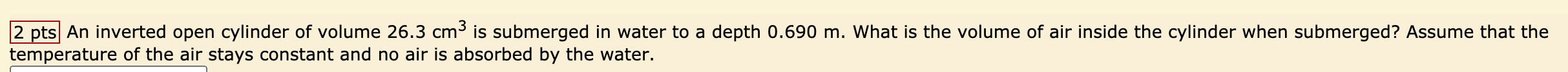2 pts An inverted open cylinder of volume 26.3 cm3 is submerged in water to a depth 0.690 m. What is the volume of air inside the cylinder when submerged? Assume that the temperature of the air stays constant and no air is absorbed by the water.

• ### A block of wood of mass 2kg floats in water, and it is noted that volume...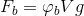A block of wood of mass 2kg floats in water, and it is noted that volume is 3/4 is submerged. density of water 1000 kg/m3. a) What is the buoyant force of the block? hint: V is volume and g is 9.81 m/s2. b) What is the density of the block?

• ### You will float a wood block in a container filled with water. Derive a formula predicting...

You will float a wood block in a container filled with water. Derive a formula predicting the percentage of the block submerged. (Hint: This is a problem in fluid statics. The weight of the block must be balanced by the buoyant force). The formula should be expressed as a ratio in terms of the volume of the block, V_block, and the volume of displaced water, V_water. The result should be given in terms of the density of the block, ρ_block,...

• ### You measure the volume of water in a graduated cylinder marked down to the tenth of...

You measure the volume of water in a graduated cylinder marked down to the tenth of a mL. It starts with a water level indicating 28 mL. You then drop an unknown object into the cylinder for a new level indication of 34.4 mL. You remove and dry the unknown object, measuring its mass to be 22.352 +/- 0.003g. a) Calculate the density of the unknown object in kg/m3 . (Density = mass/volume). Unit conversion factors are known exact quantities...